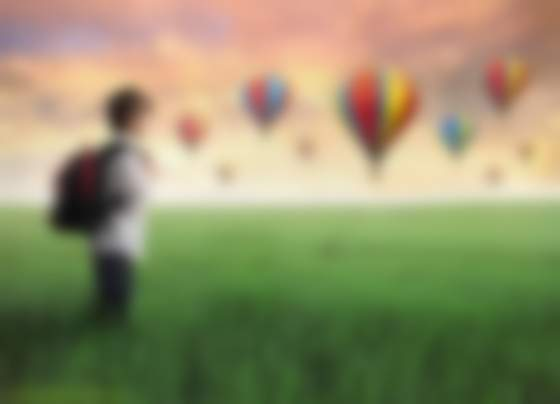# Java DIP - Applying Gaussian Filter

In this chapter, we apply Gaussian filter to an image that blurs an image. We are going to use OpenCV function GaussianBlur to apply Gaussian filter to images. It can be found under Imgproc package. Its syntax is given below −

```Imgproc.GaussianBlur(source, destination,Size,SigmaX);
```

The function arguments are described below −

Sr.No. Argument & Description
1

source

It is source image.

2

destination

It is destination image.

3

Size

It is Gaussian kernel size.

4

SigmaX

It is Gaussian kernel standard deviation in X direction.

Apart from the GaussianBlur method, there are other methods provided by the Imgproc class. They are described briefly −

Sr.No. Method & Description
1

cvtColor(Mat src, Mat dst, int code, int dstCn)

It converts an image from one color space to another.

2

dilate(Mat src, Mat dst, Mat kernel)

It dilates an image by using a specific structuring element.

3

equalizeHist(Mat src, Mat dst)

It equalizes the histogram of a grayscale image.

4

filter2D(Mat src, Mat dst, int depth, Mat kernel, Point anchor, double delta)

It convolves an image with the kernel.

5

GaussianBlur(Mat src, Mat dst, Size ksize, double sigmaX)

It blurs an image using a Gaussian filter.

6

integral(Mat src, Mat sum)

It calculates the integral of an image.

## Example

The following example demonstrates the use of Imgproc class to apply Gaussian filter to an image.

```import org.opencv.core.Core;
import org.opencv.core.CvType;
import org.opencv.core.Mat;
import org.opencv.core.Size;

import org.opencv.highgui.Highgui;
import org.opencv.imgproc.Imgproc;

public class Main {
public static void main( String[] args ) {

try {

Mat destination = new Mat(source.rows(),source.cols(),source.type());
Imgproc.GaussianBlur(source, destination,new Size(45,45), 0);
Highgui.imwrite("Gaussian45.jpg", destination);

} catch (Exception e) {
System.out.println("Error:" + e.getMessage());
}
}
}
```

## Output

When you execute the given code, the following output is seen −

### Original ImageWhen this original image is convolved with the Gaussian filter of size 11 and 45, the following output is seen.

### Gaussian filter of size 11### Gaussian filter of size 45